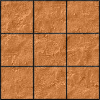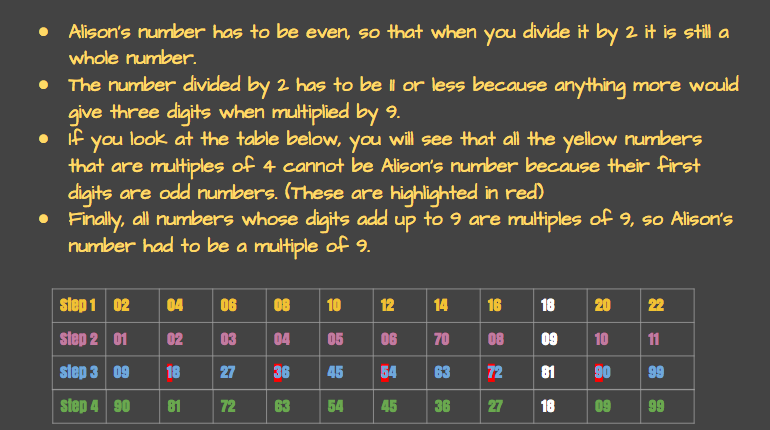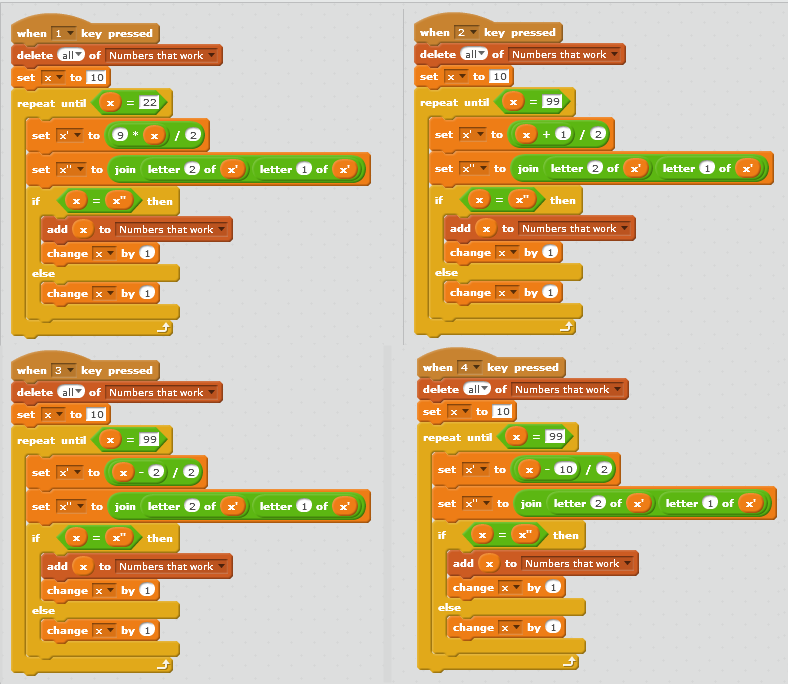#### You may also like### Pyramids

What are the missing numbers in the pyramids?### Paving the Way

A man paved a square courtyard and then decided that it was too small. He took up the tiles, bought 100 more and used them to pave another square courtyard. How many tiles did he use altogether?### Chess

What would be the smallest number of moves needed to move a Knight from a chess set from one corner to the opposite corner of a 99 by 99 square board?

# Reversals

##### Age 11 to 14 Challenge Level:

Thank you to everyone who submitted solutions to this problem, and well done to all of you!

Many of you were able to find some or all of the reversals, including Brendon, from St Mary's Catholic High School; Harry, James and Lauren, all from Beccles Free School; and Ethan, Josh, Josh and David, from Danilack Middle School, Canada.

Amy, from Wheatley Park School, sent in this excellent explanation of how she was able to find the solution to the first problem:Wayne and Jason, from ACS Barker, Singapore, explained how they found the answers for the other three parts:

Part 2

We found out that the number must be more than $21$, because $21-1=20$, and $20/2=10$, and if we try to reverse any number that is $10$ or less we will not get a two-digit number.

We found out that the number must be odd, as adding $1$ to odd numbers gives an even number and only even numbers give a whole number when divided by $2$.

We found out that the number cannot be $99$, $79$, $59$ and $39$ because adding $1$ to these numbers gives $100$, $80$, $60$ and $40$ and these numbers when divided by $2$ give $50$, $40$, $30$ and $20$ which cannot be reversed.

Within the narrowed range of numbers, we have:
• 23, 25, 27, 29
• 31, 33, 35, 37
• 41, 43, 45, 47, 49
• 51, 53, 55, 57
• 61, 63, 65, 67, 69
• 71, 73, 75, 77
• 81, 83, 85, 87, 89
• 91, 93, 95, 97
After that we just did trial and error until we found the answer. It is $73$ as $73+1=74$, $74 \div 2=37$, reverse $37$ and we get $73$, which is what we started with.

Part 3

We found out that the number must be more than $22$ because $22-2=20$ and when we divide $20$ by $2$, we would get $10$ and if we reverse a number that is $10$ or less, it does not give a two-digit number.

We found out the numbers must be even as subtracting $2$ gives an even number and only even numbers give a whole number when divided by $2$.

We found that the number cannot be $42$, $62$ or $82$, as when you subtract $2$ from them, divide the result by 2 and reverse the result, you will not get a double digit number.

Within the narrowed range of numbers, we have:
• 24, 26, 28
• 30, 32, 32, 34, 36, 38
• 40, 44, 46, 48
• 50, 52, 54, 56, 58
• 60, 64, 66, 68
• 70, 72, 74, 76, 78
• 80, 84, 86, 88
• 90, 92, 94, 96, 98
After that we did trial and error until with found the answer. It is $52$ as $52-2=50$ and $50 \div 2=25$. When you flip it around, the result you get is $52$.

Part 4

We found that the number has to be even, as only even numbers$-10$ are divisible by $2$.

We found that the number must be above $30$ as $30-10=20$, $20 \div 2=10$ and numbers less than $10$ do not have a 2-digit reverse.

We found that the number must not be $50$, $70$, $90$ as these numbers minus $10$ and divided by $2$ give $20$, $30$ and $40$, which cannot be reversed

So we start narrowing down with this range of numbers:
• 32, 34, 36, 38
• 40, 42, 44, 46, 48
• 52, 54, 56, 58
• 60, 62, 64, 66, 68
• 72, 74, 76, 78
• 80, 82, 84, 86, 88
• 92, 94, 96, 98
Trial and error again then meant we found that the number must be $62$, as $62-10=52$, $52 \div 2 = 26$ and $26$ reversed gives $62$ again.

Rachel, from NCLS Jeju in South Korea, used algebra to help her to find the solution to part 1:

If the two digit number has digits $ab$, then it can be written as $10a+b$.

Dividing by $2$ gives $\tfrac{10}{2}a+\tfrac{1}{2}b = 5a+\tfrac{1}{2}b$.

Multiplying by $9$ gives $45a + \tfrac{9}{2}b$

Since the answer is $10a+b$, when you return to the stage before reversing the digits, it becomes $10b + a$.

Since this is the same point as just after multiplying by $9$:

$45a + \tfrac{9}{2}b = 10b+a$

Simplifying this equation, it becomes:
$90a + 9b = 20b + 2a$ (multiplying by $2$)
$88a = 11b$
$8a = b$

Since $0 < a < 10$ and $0 \leq b < 10$, the only possible values of $a$ and $b$ are $a=1$ and $b=8$.
Therefore, the original number was $18$.

Sherry, also from NCLS Jeju, had this explanation for part 2:

Suppose the two-digit number is $ab$, so that it is equal to $10a+b$. When the number is reversed, it is $ba$, and is equal to $10b+a$.

From the given information, we can write the following formulae:

\begin{eqnarray}
&&\frac{10a+b+1}{2} = 10b+a\\
\implies &&10a+b+1=20b+2a\\
\implies &&8a+1 = 19b
\end{eqnarray}

Then, since $8a$ is an even number, $8a+1$ is an odd number, so $19b$ is odd, and therefore $b$ is odd.

As $a \leq 9$, $19b=8a+1\leq 73$, so $b \leq 3\tfrac{16}{19}$.

Therefore the possible values  for $b$ are $1$ and $3$.

If $b=1$, $19b=19$, so $8a=18$, which is not a multiple of $8$, so $b\neq 1$.

If $b=3$, $19b=57$, so $8a=56$ and $a=7$.

This means the starting number was $73$.

Zach solved parts 3 and 4 in a similar manner:

Part 3

From the question, if the number is $10a+b$, then:

$\frac{(10a+b)-10}{2}=10b+a$

Multiplying by $2$ gives: $10a+b-2=20b+2a$

Simplifying then gives $8a-2=19b$, so $a=5$ and $b=2$.

Since $\tfrac{1}{2}(52-2)=25$, this confirms that the number Charlie thought of was $52$.

Part 4

The initial number is $10a+b$. The question then says that $\tfrac{1}{2}((10a+b)-10)=10b+a$.

Multiplying by $2$ gives: $10a+b-10=20b+2a$

Simplifying then gives $8a-10=19b$, so $a=6$ and $b=2$.

Since $\tfrac{1}{2}(62-2)=26$, this confirms that the number Charlie thought of was $62$.

Julian, from the Britsh School, Manila, in the Philippines, used the programming language scratch to find the solutions. You can see his programs here:Both Zach and Julian were also able to solve the extension. Here is Julian's solution:

The answer will always be the last two digits of the original number reversed.

Let the second and third digits of the original number be $a$ and $b$ respectively.

The original number is $100(a+b)+10a+b = 110a+101b$

When you rotate the digits one place you get: $100a+10b+(a+b)$ = $101a+11b$

$(110a+101b)-(101a+11b)=9a+90b = 9(a+10b)$

Dividing by 9 we get $a+10b$

But the last two digits of the original number is $10a+b$!
So when you reverse the digits, you get $10b+a$.

Therefore doing this process is the same as reversing the last two digits of the original number.